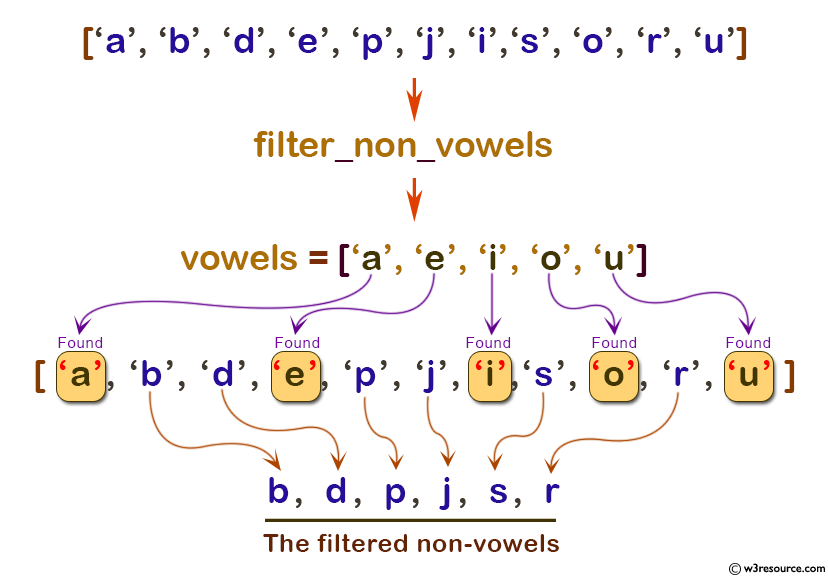# Python: filter() function

## filter() function

The filter() function construct an iterator from those elements of iterable for which function returns true.

Version:

(Python 3.2.5)

Syntax:

```filter(function, iterable)
```

Parameter:

Name Description
function A Function to be run to test if elements of an iterable returns true or false. If function is None, the identity function is assumed, that is, all elements of iterable that are false are removed.
iterable

iterable may be either a sequence, a container which supports iteration, or an iterator.

Example: Python filter() function

``````# list of alphabets
letters = ['a', 'b', 'd', 'e', 'p', 'j', 'i','s', 'o', 'r', 'u']

# function that filters non-vowels
def filter_non_vowels(letters):
vowels = ['a', 'e', 'i', 'o', 'u']

if(letters in vowels):
return False
else:
return True

filter_non_vowels = filter(filter_non_vowels, letters)

print('The filtered non-vowels are:')
for non_vowel in filter_non_vowels:
print(non_vowel)
``````

Output:

```The filtered non-vowels are:
b
d
p
j
s
r
```

Pictorial Presentation:Python Code Editor:

Previous: exec()
Next: float()

Test your Python skills with w3resource's quiz

﻿

## Python: Tips of the Day

How do I check if a list is empty?

For example, if passed the following:

```a = []
if not a:
print("List is empty")
```

Ref: https://bit.ly/2A4JXx9